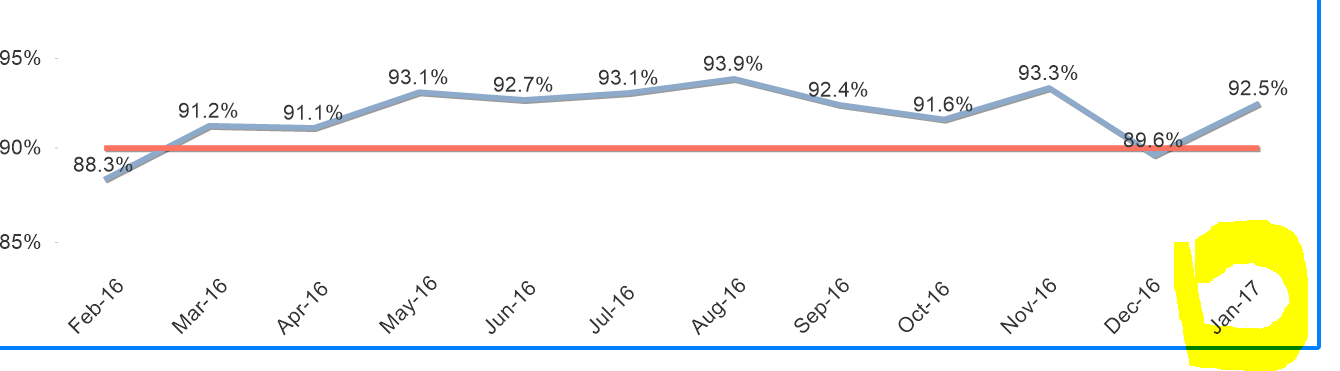# New to Qlik Sense

Discussion board where members can get started with Qlik Sense.

New Contributor III

## How to restrict the date values in Line chart X axis

Hi,

I have d date field having values  like Jan-16,Feb-16,Mar-16......................Sep17.

I want to display last 12 months of data in X-axis.

Ex: Current month is Feb 17, i have to show on x-axis from Jan-16 to Jan-17 only.

If Current month is Mar-17, i have to show data on x-axis  from Feb-16 to Feb-17.

Can any one help on this requirement.

Thanks,

Ravi

1 Solution

Accepted SolutionsMVP

## Re: How to restrict the date values in Line chart X axis

This?

num(sum({<Surface={'Total Room'}, CalendarDate = {"\$(='>=' & Date(AddYears(Max(CalendarDate), -1), 'MMM-YY') & '<=' & Date(Max(CalendarDate), 'MMM-YY'))"}>}Pass)/(sum({<Surface={'Total Room'}>}Fail)+ sum({<Surface={'Total Room'}>}Pass)+sum({<Surface={'Total Room'}>}Caution)),'#,##0.0%')

9 RepliesPartner

## Re: How to restrict the date values in Line chart X axis

First create MonthSerial like this.

Year * 12  + Month

Here is how to do it in script

MonthMap:

Name,Num

Jan,1

Feb,2

.

.

Dec,12

];

Assuming field name of d date is d_date

ApplyMap('MonthMap',left(d_date,3)) + (right(d_date,2)*12) as MonthSerial

Then in expression of Measure use set analysis like this

Sum( {<MonthSerial={">=\$(=max(MonthSerial)-12) <\$(=max(MonthSerial))"}>} Sales)

New Contributor III

## Re: How to restrict the date values in Line chart X axis

Hi ,

Is there any way to restrict by using calculated dimension in the chart.Partner

## Re: How to restrict the date values in Line chart X axis

You cannot use calculated dimension in Set Analysis and Set analysis is the only way you restrict data,

I'll need a sample app to do that without scripting.

New Contributor III

## Re: How to restrict the date values in Line chart X axis

Sample.qvw

Hi Shubham,

Can you check the Sample file.MVP

## Re: How to restrict the date values in Line chart X axis

This?

num(sum({<Surface={'Total Room'}, CalendarDate = {"\$(='>=' & Date(AddYears(Max(CalendarDate), -1), 'MMM-YY') & '<=' & Date(Max(CalendarDate), 'MMM-YY'))"}>}Pass)/(sum({<Surface={'Total Room'}>}Fail)+ sum({<Surface={'Total Room'}>}Pass)+sum({<Surface={'Total Room'}>}Caution)),'#,##0.0%')

New Contributor III

## Re: How to restrict the date values in Line chart X axis

Thanks Sunny,

Its working.

New Contributor III

## Re: How to restrict the date values in Line chart X axis

Hi Sunny,

This expression is working for last 12 months.

I am having calendar dates from Jan-16 to Sep-17,so it is showing data for  Oct-16 to Sep 17.

My requirement is ,it suppose to show Feb-16 to Jan-17.

If Calendar date is Mar-17 ,then my o/p is Mar-16 to Feb-17.

Can you help on this requirement.

Thanks,

RaviMVP

## Re: How to restrict the date values in Line chart X axis

Calendar date is Jan-16 to Sep-17 and you want to see from Feb-16 to Jan-17? What is the logic here? Can you explain it?

New Contributor III

## Re: How to restrict the date values in Line chart X axis

My Chart always should show the data of last 12 Months.

Ex:Current month is Feb 17, so my chart should display data from Feb-16 to  Jan-17.

If i reload  the report in March-17, the chart must have data from Mar-16 to Feb-17.

If i  reload the report in Apr-17,the chart must have data from Apr-16 to Mar-17.The highlighted month always be [Current month-1 ] and total months in the graph are 12.